# ski-in / ski-out - Page 25

Viewing mode
708 results
• Slopes access :
• ski-in (-50 m)
• Rooms :
• Studio
• Studio + cabin(s)
• Total surface (sq.m) :
• 31 sq.m
•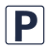•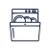•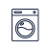•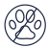• Slopes access :
• 50 -300m to the slopes
• Rooms :
• 2 rooms
• 2 rooms + cabin(s)
• Total surface (sq.m) :
• 60 sq.m
•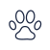•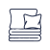••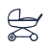• Slopes access :
• 50 -300m to the slopes
• Rooms :
• 2 rooms
• Total surface (sq.m) :
• 22 sq.m
• Garage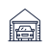••••• Slopes access :
• 50 -300m to the slopes
• Rooms :
• Studio
• Studio + cabin(s)
• Total surface (sq.m) :
• 27 sq.m
•••• Slopes access :
• ski-in (-50 m)
• Rooms :
• Duplex
• Total surface (sq.m) :
• 50 sq.m
•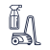•••• Slopes access :
• ski-in (-50 m)
• Total surface (sq.m) :
• 41 sq.m
••••• Slopes access :
• ski-in (-50 m)
• Rooms :
• Studio
• Studio + cabin(s)
• Total surface (sq.m) :
• 30 sq.m
••••• Slopes access :
• ski-in (-50 m)
• Rooms :
• 2 rooms
• Total surface (sq.m) :
• 43-54 sq.m
•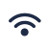••••••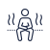•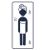• Slopes access :
• 50 -300m to the slopes
• Rooms :
• 2 rooms
• 2 rooms + cabin(s)
• Total surface (sq.m) :
• 47 sq.m
••••• Slopes access :
• ski-in (-50 m)
• Rooms :
• 3 rooms
• Total surface (sq.m) :
• 60 sq.m
••• Garage•••••• Slopes access :
• 50 -300m to the slopes
• Rooms :
• 3 rooms
• Total surface (sq.m) :
• 62 sq.m
••• Slopes access :
• 50 -300m to the slopes
• Rooms :
• Studio
• Studio + cabin(s)
• Total surface (sq.m) :
• 26 sq.m
••• Slopes access :
• ski-in (-50 m)
• Rooms :
• 3 rooms
• Total surface (sq.m) :
• 55 sq.m
•••••• Slopes access :
• 50 -300m to the slopes
• Rooms :
• 3 rooms
• Total surface (sq.m) :
• 45 sq.m
••••• Slopes access :
• 50 -300m to the slopes
• Rooms :
• 4 rooms
••••• Slopes access :
• 50 -300m to the slopes
• Rooms :
• Studio
• Studio + cabin(s)
• Total surface (sq.m) :
• 25 sq.m
••• Slopes access :
• ski-in (-50 m)
• Total surface (sq.m) :
• 48 sq.m
••••• Slopes access :
• ski-in (-50 m)
• Rooms :
• Studio
• Studio + cabin(s)
• Total surface (sq.m) :
• 30 sq.m
••• Slopes access :
• ski-in (-50 m)
• Rooms :
• Studio
• Studio + cabin(s)
• Total surface (sq.m) :
• 26 sq.m
•• Slopes access :
• ski-in (-50 m)
• Rooms :
• Studio
• Studio + cabin(s)
• Total surface (sq.m) :
• 34 sq.m
•••